Data Structures and Algorithms with Object-Oriented Design Patterns in C### More Big Oh Fallacies and Pitfalls

The purpose of this section is to dispel some common misconceptions about big oh. The next fallacy is related to the selection of the constants c and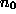used to show a big oh relation.

Fallacy  Consider non-negative functions f(n),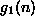, and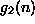, such that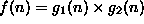. Since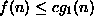for all integersif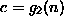, then by Definition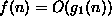.

This fallacy often results from the following line of reasoning: Consider the function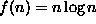. Let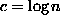and. Then f(n) must be O(n), since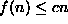for all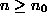. However, this line of reasoning is false because according to Definition, c must be a positive constant, not a function of n.

The next fallacy involves a misunderstanding of the notion of the asymptotic upper bound.

Fallacy  Given non-negative functions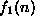,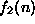,, and, such that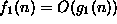,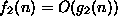, and for all integers,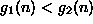, then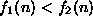.

This fallacy arises from the following line of reasoning. Consider the function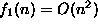and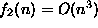. Since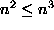for all values of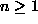, we might be tempted to conclude that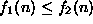. In fact, such a conclusion is erroneous. For example, consider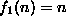and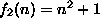. Clearly, the former is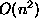and the latter is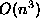. Clearly too,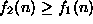for all values of!

The previous fallacy essentially demonstrates that while we may know how the asymptotic upper bounds on two functions are related, we don't necessarily know, in general, the relative behavior of the two bounded functions.

This fallacy often arises in the comparison of the performance of algorithms. Suppose we are comparing two algorithms, A and B, to solve a given problem and we have determined that the running times of these algorithms are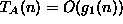and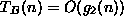, respectively. Fallacydemonstrates that it is an error to conclude from the fact that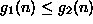for allthat algorithm A will solve the problem faster than algorithm B for all problem sizes.

But what about any one specific problem size? Can we conclude that for a given problem size, say, that algorithm A is faster than algorithm B? The next fallacy addresses this issue.

Fallacy  Given non-negative functions,,, and, such that,, and for all integers,, there exists an integerfor which then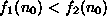.

This fallacy arises from a similar line of reasoning as the preceding one. Consider the functionand. Sincefor all values of, we might be tempted to conclude that there exists a valuefor which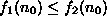. Such a conclusion is erroneous. For example, consider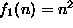and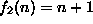. Clearly, the former isand the latter is. Clearly too, sincefor all values of, there does not exist any value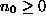for which.

The final fallacy shows that not all functions are commensurate :

Fallacy  Given two non-negative functions f(n) and g(n) then either f(n)=O(g(n)) or g(n)=O(f(n)).

This fallacy arises from thinking that the relation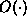is like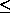and can be used to compare any two functions. However, not all functions are commensurate.Consider the following functions: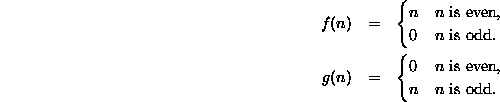Clearly, there does not exist a constant c for which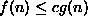for any even integer n, since the g(n) is zero and f(n) is not. Conversely, there does not exist a constant c for which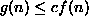for any odd integer n, since the f(n) is zero and g(n) is not. Hence, neither f(n)=O(g(n)) nor g(n)=O(f(n)) is true.Copyright © 2001 by Bruno R. Preiss, P.Eng. All rights reserved.